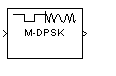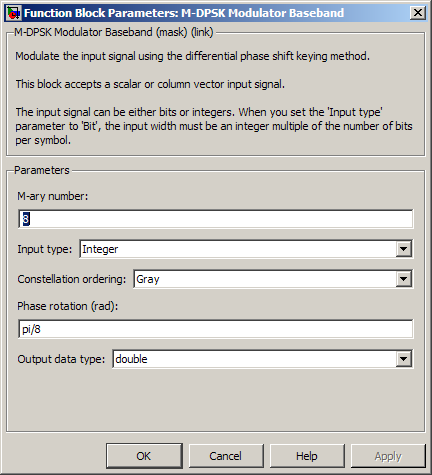M-DPSK Modulator Baseband

Modulate using M-ary differential phase shift keying method

Library

PM, in Digital Baseband sublibrary of Modulation

•Description

The M-DPSK Modulator Baseband block modulates using the M-ary differential phase shift keying method. The output is a baseband representation of the modulated signal. The M-ary number parameter, M, is the number of possible output symbols that can immediately follow a given output symbol.

The input must be a discrete-time signal. For integer inputs, the block can accept the data types int8, uint8, int16, uint16, int32, uint32, single, and double. For bit inputs, the block can accept int8, uint8, int16, uint16, int32, uint32, boolean, single, and double.

The input can be either bits or integers, which are binary-mapped or Gray-mapped into symbols.

This block accepts column vector input signals. For a bit input, the input width must be an integer multiple of the number of bits per symbol.

Integer-Valued Signals and Binary-Valued Signals

If you set the Input type parameter to Integer, then valid input values are integers between 0 and M-1. In this case, the input can be either a scalar or a frame-based column vector. If the first input is k1, then the modulated symbol is

$\mathrm{exp}\left(j\theta +j2\pi \frac{{k}_{1}}{m}\right)$

where θ represents the Phase rotation parameter. If a successive input is k, then the modulated symbol is

When you set the Input type parameter to Bit, the block accepts binary-valued inputs that represent integers. The block collects binary-valued signals into groups of K = log2(M) bits

where

K represents the number of bits per symbol.

The input vector length must be an integer multiple of K. In this configuration, the block accepts a group of K bits and maps that group onto a symbol at the block output. The block outputs one modulated symbol for each group of K bits.

The input can be a column vector with a length that is an integer multiple of K.

In binary input mode, the Constellation ordering parameter indicates how the block maps a group of K input bits to a corresponding phase difference. The Binary option uses a natural binary-to-integer mapping, while the Gray option uses a Gray-coded assignment of phase differences. For example, the following table indicates the assignment of phase difference to three-bit inputs, for both the Binary and Gray options. θ is the Phase rotation parameter. The phase difference is between the previous symbol and the current symbol.

Current InputBinary-Coded Phase DifferenceGray-Coded Phase Difference
[0 0 0]
[0 0 1] jθ + jπ/4 jθ + jπ/4
[0 1 0] jθ + jπ2/4 jθ + jπ3/4
[0 1 1] jθ + jπ3/4 jθ + jπ2/4
[1 0 0] jθ + jπ4/4 jθ + jπ7/4
[1 0 1] jθ + jπ5/4 jθ + jπ6/4
[1 1 0] jθ + jπ6/4 jθ + jπ4/4
[1 1 1] jθ + jπ7/4 jθ + jπ5/4

For more details about the Binary and Gray options, see the reference page for the M-PSK Modulator Baseband block. The signal constellation for that block corresponds to the arrangement of phase differences for this block.

Dialog BoxM-ary number

The number of possible output symbols that can immediately follow a given output symbol.

Input type

Indicates whether the input consists of integers or groups of bits. If this parameter is set to Bit, then the M-ary number parameter must be 2K for some positive integer K.

Constellation ordering

Determines how the block maps each group of input bits to a corresponding integer.

The phase difference between the previous and current modulated symbols when the input is zero.

Output data type

The output data type can be either single or double. By default, the block sets this to double.

Supported Data Types

PortSupported Data Types

Input

• Double-precision floating point

• Single-precision floating point

• Boolean (binary input mode only)

• 8-, 16-, and 32-bit signed integers

• 8-, 16-, and 32-bit unsigned integers

Output

• Double-precision floating point

• Single-precision floating point

Pair Block

M-DPSK Demodulator Baseband

References

 Pawula, R. F., "On M-ary DPSK Transmission Over Terrestrial and Satellite Channels," IEEE Transactions on Communications, Vol. COM-32, July 1984, 752-761.

Extended Capabilities

C/C++ Code GenerationGenerate C and C++ code using Simulink® Coder™.

Introduced before R2006a